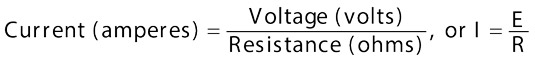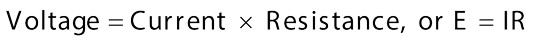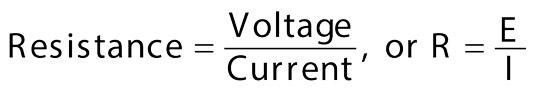##### 2022 / 2023 ASVAB For DummiesYou will need to brush up on your knowledge of electrical resistance for the ASVAB Electronic Information subtest. Current doesn’t just flow in any properly working circuit unimpeded. Resistance pops up along the way. If the flow of electricity needs to be regulated, resistance is deliberately set up in a circuit.

If the flow weren’t regulated, the motors powering devices like can openers and microwave ovens would quickly overheat and melt. (But before that happens, hopefully a fuse would blow or a circuit breaker would trip, halting current flow and saving the equipment.) In a sense, even a wire, such as a filament in a light bulb, is a type of resistance and is a way to deliberately create circuit resistance.

Sometimes a circuit must be opened in order to add or remove resistance. In other words, the flow of the electricity must be interrupted in order to physically change the resistance. Using a circuit breaker, which is a device that automatically interrupts the electrical current, is an example of opening a circuit to control the current. When the circuit breaker trips, the electrical device can no longer operate.

Some devices use a rheostat, which can vary the resistance without opening the circuit — the device can continue to work even as the resistance is altered. If an application doesn’t use all the electricity, the rheostat absorbs it. A dimmer switch on a light is an example of a rheostat. You increase the amount of resistance to dim the light and decrease the resistance to brighten the light.

## Ohm’s law: Relating resistance to current and voltage

The amount of resistance that interferes with the flow is measured in ohms (pronounced just like those yoga chants). The symbol for ohm is the Greek letter omega, which looks like an upside-down horseshoe: Ω. Resistance can be measured by dividing the voltage measured at any given point (the voltmeter reading) by the amount of current at the same point in a circuit (the ammeter reading). Or you can measure the resistance directly by an ohmmeter.

If you have a current flowing through a wire, three influences are present:

• The amount of voltage, measured in volts

• The resistance to the current, measured in ohms

• The amount of current, measured in amps

These three units are always present in a specific relationship to each other. If you know the value of any two of the influences, you can find the value of the third. (Yes, this requires more math. Sorry.)

Ohm’s law, which was first stated by Georg Simon Ohm, reads, “The current in a circuit is directly proportional to the applied voltage and inversely proportional to the circuit resistance,” but it’s actually easier to understand in mathematical terms. When stating the relationship mathematically, abbreviations are used, where I is current, E is voltage, and R is resistance:This essentially means that current in a basic circuit is always dependent on the voltage and resistance in the circuit. If you use a higher-voltage battery (increase E), the resistance doesn’t change, but current in the circuit increases. By the same token, if you leave the same battery in the circuit but increase the resistance (increase R), current decreases.

Here are two other ways to write the same formula, solved for voltage and resistance:Ohm’s law works exactly the same, no matter which format you use.